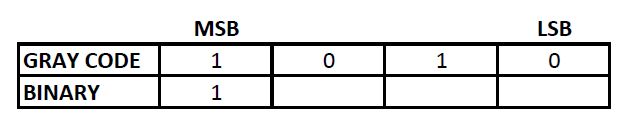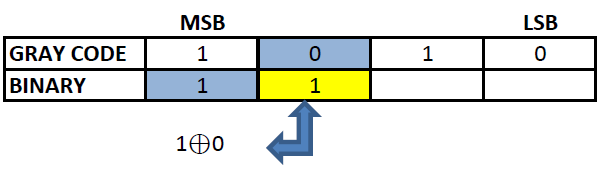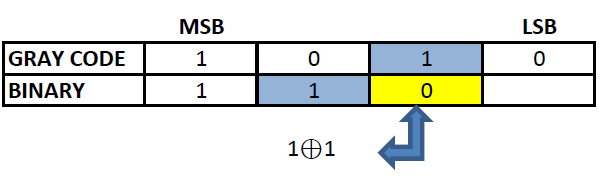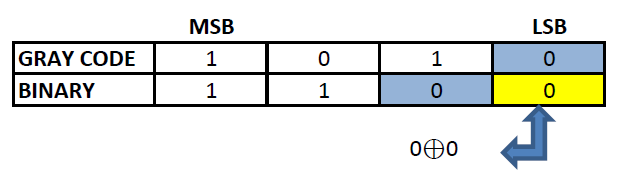# How to convert gray code to octal

## Gray code

Gray code, also known as reflected binary code, is a code having digits 0 and 1. Gray code do not have place value for its digits. Any successive codes in Gray code system have only one bit changes.

## Octal Number

Octal numbers use digits from 0-7 only. It is known as base-8 number. The place value of each digits of an octal number varies as the whole number powers of 8 starting from the right (Least Significant Digit). The first single digit number in octal system is 0 and the last is 7. Similarly, the first two digit octal number is 10 and the last is 77 and so on. Octal number system was widely used in early computers.

## Binary number

Binary numbering system uses only two symbols 0 and 1. Each digits of a binary number is referred to as bits. Binary system is also known as base -2 system. Each digit is represented by the increasing power of 2 from the LSB (Least Significant Bit). Binary system is the heart of digital electronics and is used for information flow. In digital electronics, 0 and 1 are used to denote logic states, high and low. Arithmetic operations are also possible in binary system.

## Gray code to octal conversion

Gray code cannot be directly converted to octal. For converting gray code to octal, we have to convert the gray code to binary first and then convert the binary code to octal.
Gray to octal conversion can be well understood with an example.

### Let us convert 1010 from gray code to octal value:

To convert 1010 to octal, we have to convert 1010 to binary first.
Step 1: The MSB (Most Significant Bit) of a binary and gray code will be the same.Step 2: The next digit of binary will be the EXOR of the MSB of binary and the second MSB of the gray code.Step 3: Similarly EXOR the digit obtained in the previous step and the third MSB of gray code to obtain the third MSB of binary.Step 4: Repeat the previous step till the LSB of gray code is found.### The Binary corresponding to gray code 1010 is 1100. Now this binary code can be converted to octal.

11002 = 148
Therefore, Gray code 1010 = 148